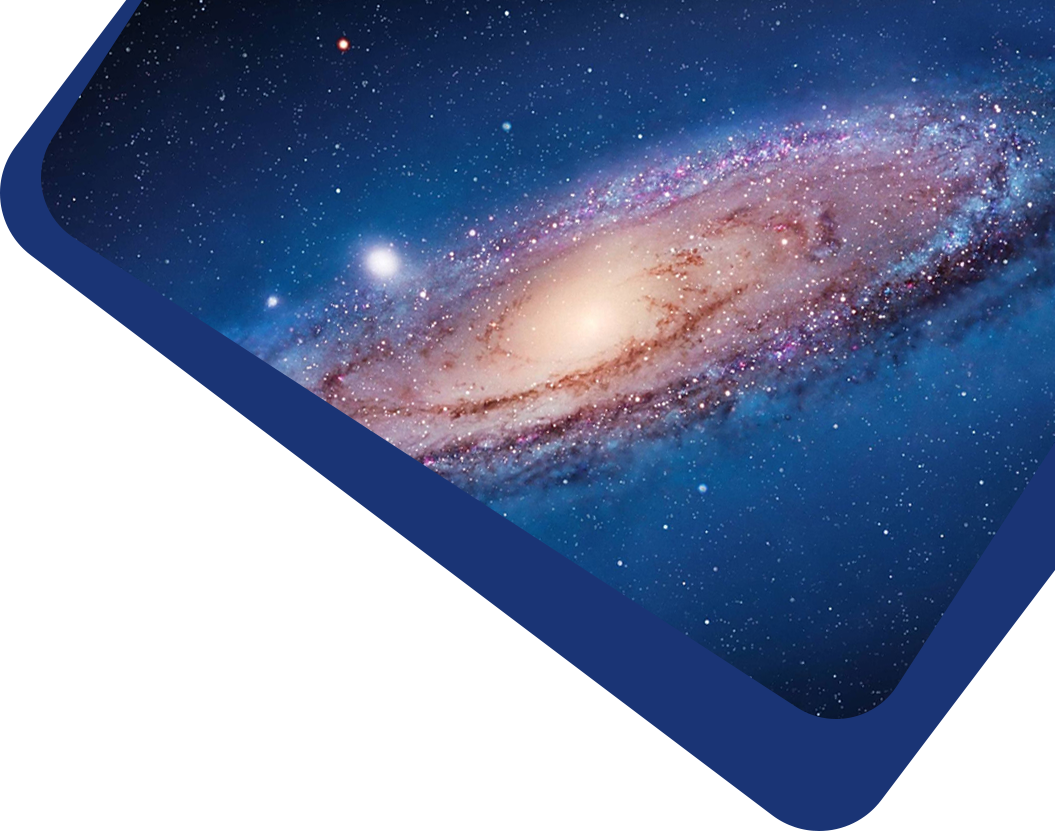Highlights

## Photometric Redshift Estimates using Bayesian Neural Networks in the CSST Survey

#### Xingchen Zhou, Yan Gong, Xian-Min Meng, Xuelei Chen, Zhu Chen et al.

Abstract

Galaxy photometric redshift (photoz) is crucial in cosmological studies, such as weak gravitational lensing and galaxy angular clustering measurements. In this work, we try to extract photoz information and construct its probability distribution function (PDF) using the Bayesian neural networks from both galaxy flux and image data expected to be obtained by the China Space Station Telescope (CSST). The mock galaxy images are generated from the Hubble Space Telescope - Advanced Camera for Surveys (HST-ACS) and COSMOS catalogs, in which the CSST instrumental effects are carefully considered. In addition, the galaxy flux data are measured from galaxy images using aperture photometry. We construct a Bayesian multilayer perceptron (B-MLP) and Bayesian convolutional neural network (B-CNN) to predict photoz along with the PDFs from fluxes and images, respectively. We combine the B-MLP and B-CNN together, and construct a hybrid network and employ the transfer learning techniques to investigate the improvement of including both flux and image data. For galaxy samples with signal-to-noise ratio (SNR) > 10 in g or i band, we find the accuracy and outlier fraction of photoz can achieve σNMAD = 0.022 and η = 2.35% for the B-MLP using flux data only, and σNMAD = 0.022 and η = 1.32% for the B-CNN using image data only. The Bayesian hybrid network can achieve σNMAD = 0.021 and η = 1.23%, and utilizing transfer learning technique can improve results to σNMAD = 0.019 and η = 1.17%, which can provide the most confident predictions with the lowest average uncertainty.

Keywords

(cosmology:) large-scale structure of universe – methods: statistical – techniques: image processing

Full Text
Refbacks